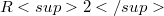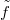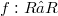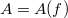﻿

### Note on planar functions over the reals

#### Abstract

The following construction was used in a paper of Kárteszi  illustrating the role of Cremona transformations for secondary school students.This is a typical construction in the theory of flat affine planes, see Salzmann , Groh  and due to Dembowski and Ostrom  for the case of finite ground fields. Let$R2$ be the classical euclidean affine plane and$\tilde{f}$ be the graph of a real function$f : R → R$ (R denotes the field of real numbers).Define a new incidence structure$A = A(f)$ on the points of$R2$ in which the new lines are the vertical lines of$R2$ and the translates of$\tilde{f}$.The incidence is the set-theoretical element of relation. (For the definition of incidence structure,affine plane etc. we refer to Dembowski ).

DOI Code: 10.1285/i15900932v10n1p59

Full Text: PDF